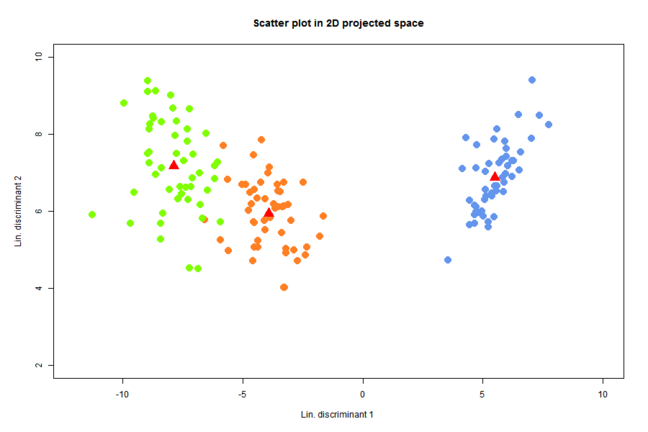## Want to keep learning?

This content is taken from the Partnership for Advanced Computing in Europe (PRACE)'s online course, Managing Big Data with R and Hadoop. Join the course to learn more.
3.11

## Partnership for Advanced Computing in Europe (PRACE)Scatter plot of 3 groups and their centroids

# Example 1: Computing groups centroids

## Computing centroids of big data

In this example we demonstrate how to compute groups centroids using mapreduce from rmr2. We consider the data about customers of CEnet, stored in dfs as /CEnetBig. The first 10 rows of the data are as follows:

## Data

         id 2016_1 2016_2 2016_3 2016_4 2016_5 2016_6 2016_7 2016_8 2016_9 2016_10 2016_11 2016_12 type
[1,] 100373 137.66 141.57 128.83 133.00  97.39 116.62 123.97 156.83  90.50   98.62  118.61  152.34    4
[2,] 100194  98.32 119.40 120.30 105.67  90.26  80.13  80.62 108.63 104.30  123.31  101.93  140.85    2
[3,] 100565 127.60 133.79  90.15  62.33  87.96  92.20  72.04 113.69  65.95   82.69   85.72  121.81    2
[4,] 100553 154.60 175.10  94.64 123.41 116.96  94.57 124.25 138.89  72.57  121.03  122.09  106.79    4
[5,] 100902 162.26 157.10 114.03 145.30 144.44  73.91 131.93 142.66 125.98   92.90  104.70  161.60    5
[6,] 100883 119.66 148.39 144.38 105.61  66.66  70.84 110.15 114.50  75.60   85.22  125.67   90.76    2
[7,] 100352 147.50 110.85  95.61  77.76  98.78  54.88 104.35  53.52  73.09  101.75   77.65   58.19    1
[8,] 100863 108.84  75.53 105.55  82.24 119.41  49.98  94.74 136.62 101.14   71.08   29.29  131.81    2
[9,] 100626 109.20 107.59  96.95  88.14  94.12  80.71  68.83  87.45  66.52   95.28   83.21   82.38    1
[10,] 100867 114.71  88.94  88.45  75.03  74.58  55.55 126.48  42.78  88.01  124.90  137.59  152.55    2


In the first column we have the id of the customer, in the next 12 columns we have the values of their bills for the months January-December 2016 and the last column (type) contains the data about the product (package) that the customer has. This column defines 5 groups for which we compute the centroids (the 12 dimensional vectors with the mean values of columns 2016_1 - 2016_12).

First we load our data. The data is already stored in the HDFS (you got it with the virtual machine that you installed in Week 1). We have a full data file (CEnetBig) that we can load by:

CEnetBig=from.dfs("/CEnetBig")


However, we try to avoid calling it directly, but rather pass it directly to the map-reduce function (see below).

## Method

Note that the data chunks containing our data have all 5 groups. Therefore, we compute the centroids of these groups with map-reduce as follows.

### Map

For data chunk i we compute via the map function the i-th group sum s_1i, s_2i,...,s_5i and the corresponding group sizes: n_1i,n_2i,...,n_5i. The map function therefore returns key-value pairs (k,{n_ki, s_ki}), where k is the group label (1,2,…,5), n_ki is the number of data rows in k-th group, while s_ki is the sum of all the data rows from the k-th group.

mapper = function (., X) {
n=nrow(X);
ones=matrix(rep(1,n),nrow=n,ncol=1);
ag=aggregate(cbind(ones,X[,2:13]),by=list(X[,14]),FUN="sum")
key=factor(ag[,1]);
keyval(key,split(ag[,-1],key))
}


Comment of the mapper code:

Line Result
n=nrow(X) gives the number of rows in X, i.e.,
from CEnetBig with 1000000 rows
ones=matrix(rep(1,n),nrow=n,ncol=1) creates a nx1 matrix with 1 values, which is used as a counter during aggregation
ag=aggregate(cbind(ones,X[,2:13]),
by=list(X[,14]),FUN=”sum”)
- adds the values of each row grouped by type (column 14)
- ag is a 5x14 matrix:
(type, count, 2016_1,…, 2016_12)
- cbind(ones,X[,2:13]): combines matrix ones with the columns 2:13 from X
key=factor(ag[,1]) key is a list from 1 to 5
keyval(key,split(ag[,-1],key)) - creates a key-value object (a collection of key-value pairs) from two R objects, extract keys or values from a key value object or concatenate multiple key value objects
- ag[,-1]: indicates all columns but the first one
- split(ag[,-1],key): separates ag by key

### Reduce

The REDUCE part computes the final sums of the data rows for each group and returns the key-value pairs (k,{n_ki, s_ki}) for the whole dataset.

reducer = function(k, A) {
keyval(k,list(Reduce('+', A)))
}


### Map-reduce

Once we have defined the map and reduce function we compute the group sums with mapreduce.

GroupSums <-   from.dfs(
mapreduce(
input = "/CEnetBig",
map = mapper,
reduce = reducer,
combine = T
)
)


## Final code

Finally, we compute the group centroids by taking the values of the key-value pairs. Note that the first entry of each value is the group size and the rest of the entry is the row representing the group sums. To obtain the centroids we divide each row of the group sums with the size of the group.

GroupSumsM <- matrix(unlist(GroupSums\$val), ncol =  13, byrow  = TRUE)
Centroids<-GroupSumsM[,-1]/GroupSumsM[,1]


If you run the code you should obtain the following results:

> Centroids
[,1]     [,2]      [,3]      [,4]      [,5]      [,6]      [,7]      [,8]      [,9]     [,10]     [,11]    [,12]
[1,] 109.9310 104.9678  94.98656  80.08108  80.00087  64.97981  84.93587  90.07869  70.00322  79.85767  89.94883 100.0320
[2,] 120.0249 115.0168 104.95868  89.96294  89.97693  74.99977  95.04289 100.05404  80.02519  90.05004  99.99359 109.9933
[3,] 130.0478 125.0739 115.14003 100.00783  99.90976  85.01050 105.03596 110.00461  90.08632 100.01509 110.03724 120.0029
[4,] 139.9501 135.0315 124.96959 110.03113 109.99999  94.94679 114.93834 120.03891 100.01716 110.02524 119.95535 129.9921
[5,] 149.9407 145.0123 135.06575 119.97880 120.06271 105.05000 124.97573 129.95944 109.92863 120.02247 130.02287 139.9866